The Euler Number

Next: Symmetry and orbifolds Up: Geometry and the Imagination Previous: Summary

# The Euler Number

If we have a polyhedron, we can compute its Euler number,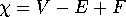. In fact, we computed Euler numbers ad delectam. Why did we do this? One reason is that they are is easy to compute. But that is not obviously a compelling reason for doing anything in mathematics. The real reason is that it is an invariant of the surface (it does not depend upon what map one puts on the surface) and because it is connected to a whole array of other properties a surface might have that one might notice while trying to describe it.

## Descartes's Formula

One easy example of this is Descartes' formula. If one looks at a polyhedral surface and makes a naive attempt to describe it visually, one might try to describe how pointy the surface is. A more sophisticated way to describe how pointy a surface is at a vertex is to compute the angular defect at the vertex, that is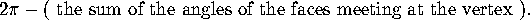When we investigated how pointy a polyhedron was, summing over all of the vertices to obtain the total angular defect, we discovered that there was a direct connection between pointyness and Euler Number: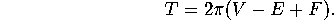## The Gauss Map (Flashlights)

Although projecting Conway's image onto the celestial sphere was fun, again it was not in and of itself a mathematically valuable exercise. The point was to get a feel for the Gauss map. The Gauss map is used to project a surface onto the celestial sphere. For a polyhedron, we saw that, if one traced a path that remained on a flat face, the Gauss image of that path was really a point. We saw that if we traced a path that went around a vertex, the Gauss image was a spherical polygon. If three edges met at the given vertex, the Gauss image traced out a spherical triangle whose interior could be thought of as the image of that vertex. Moreover, the angles of the triangle were the supplements of the vertex angles. Using the formula for the area of a spherical triangle, namely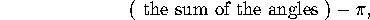if the vertex angles were, the area of the Gauss image of a path around the vertex would be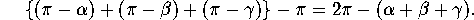The right hand side of this formula is just the angular defect at the vertex. Thus if we add up the areas of the images of path about all of the vertices, we obtain the total defectof the original surface. Since no other parts of the image contribute to the area, we have shown that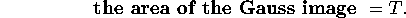Exploiting the earlier connection, we can also sayThis is known as the Gauss-Bonnet formula.

## Curvature (Kale and cabbage)

Again, cutting up kale and cabbage was fun and the tape of Thurston and Conway sticking potato peel to the chalkboard will become a classic, but there was a serious mathematical purpose behind it. If one looks at a surface and wants to try to describe it visually, one might want to describe it by telling how curly it is. While the surface of a cylinder, for example, does not look visually as though it curves and bends very much, the surface of a trumpet does. Peeling a surface, that is, removing a thin strip from around a portion of the surface and then seeing how much the angle between the ends of the strip opens up (or closes around) as it is laid flat quantifies the curviness of the portion of the surface surrounded by the strip. Mathematically, this is called the integrated curvature of that portion of the surface.

When we sum over portions that amount to the whole surface, we get the total Gaussian curvature of the surface.

### Curvature for Polyhedra

Lets apply these ideas to a polyhedron. In particular, we might consider a strip of polyhedron peel that just goes around one vertex of a polyhedron. Then we would find that the path opens up by an angle equal to the defect at that vertex, and so for such a path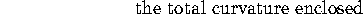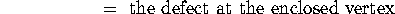For a path that goes around several vertices the curvature is the sum of the defects of all the surrounded vertices. Thus for a polyhedron,### Curvature on surfaces

To pass from a polyhedral surface to a smooth surface and to define curvature with mathematical precision, one needs to use integration in the definition for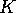. But the conceptual idea is still the same. Any curved surface can be approximated by a polyhedral one with lots and lots of vertices. The curvature of the surface within a path (a smooth piece of peel) is then very nearly equal to the sum of the defects at all the encircled vertices. By a technical limiting argument that involves integrals to give a precise meaning to curvature,, we find that for any surface## Discussion

• There are many other connections between these four concepts. Can you suggest any more? (This is also a discussion question for the gang of four.)
• The number of handles on a surface is another visual characteristic. How does this relate to the total curvature?

Next: Symmetry and orbifolds Up: Geometry and the Imagination Previous: Summary

Peter Doyle